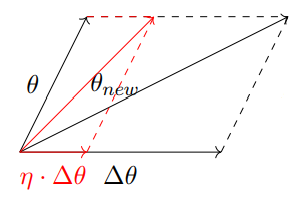# Math behind the Gradient Descent Algorithm

## Why do we care about the Gradient descent algorithm?

As we know, supervised machine learning algorithms are described as learning a target function (f) that best maps input variables (x) to an output variable (Y).

## Gradient Descent Algorithm: An Explanation

Gradient descent algorithm is an optimization technique, that follows the negative gradient of an objective function in order to locate the minimum of a function. It is technically called the first-order optimization algorithm as it explicitly makes use of the first-order derivative of the target objective function. Functioning of GDA is represented can be visualized as below:

## Why does the algorithm HAVE to follow the NEGATIVE gradient of the loss function?

To find a local minimum of a function using gradient descent, we take steps proportional to the negative of the gradient of the function at the current point. In other words, we move in the direction opposite to the gradient. Have you ever wondered why?Source: Deep Learning IIT Ropar — Mitesh Khapra

--

--